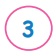Home | | Maths 3rd Std | Collecting and Representing data

# Collecting and Representing data

Symbols and pictures can be used to represent data. This is known as Pictorial Representation. This helps us to study and understand data easily.

Collecting and Representing data

Pictorial Representation

Symbols and pictures can be used to represent data. This is known as Pictorial Representation. This helps us to study and understand data easily.

Example :

Look at the below picture and fill the required data.1. How manyare there?2. How manyare there?3. Circle the animal which is more in count.?4. Circle the animal which is less in count?5. What is the difference between highest and lowest count?6. Total number of animals in the zoo train is.

Example :

The following picture represents the data on fruits sold in a shop.Look at the above picture and fill the required data.

1. Name the fruit which was sold more?

2. Name the fruit which is sold less. Orange

3. Find the number of apples sold.

4. Write the number of bananas sold?

5. what is the difference in the number of pineapples sold and oranges sold ?

Practice

1. Collect the data from 40 of your friends about their favourite food and represent it in Picture.2. The following picture represents the number of chocolates sold at a shop in a week.Answer the following questions from the data given in the above table.

1. The total number of chocolates sold on Thursday is 40

2. The sale was maximum on Monday

3. The sale was minimum on Saturday

4. Sales were equal on and Tuesday and Friday

5. The total number of chocolates sold in six days are 180 Chocolates.

Tags : Information Processing | Term 1 Chapter 6 | 3rd Maths , 3rd Maths : Term 1 Unit 6 : Information Processing
Study Material, Lecturing Notes, Assignment, Reference, Wiki description explanation, brief detail
3rd Maths : Term 1 Unit 6 : Information Processing : Collecting and Representing data | Information Processing | Term 1 Chapter 6 | 3rd Maths